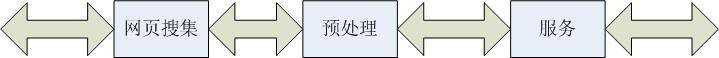2015/05/13 02:05

# 一、搜索引擎

1）搜索引擎的工作原理

－三段式工作流程2）搜索引擎算法

3）搜索引擎排名算法分类

# 二、HITS算法

1）Hits算法的基本思想

1.好的Hub型网页指向好的Authority网页

2.好的Authority网页是由好的Hub型网页所指向的网页。

2）Hits算法

HITS(Hyperlink - Induced Topic Search) 算法是利用HubPAuthority的搜索方法,具体算法如下:

1.S中的网页数量较少

2.S中的网页是与查询q相关的网页

3.S中的网页包含较多的权威（Authority）网页

V1 中的网页到V2 中的网页的超链接为边集E ,形成一个二分有向图. 对V1 中的任一个顶点v ,用h ( v) 表示网页v 的Hub 值,且h ( v)收敛;对V2 中的顶点u ,用a ( u) 表示网页的Authority 值。

开始时h ( v) = a ( u) = 1 ,对u 执行I 操作,修改它的a ( u) ,对v执行O操作,修改它的h ( v) ,然后规范化a ( u)Ph ( v) ,如此不断的重复计算下面的I操作和O操作,直到a ( u) 。

HITS算法可以获得比较好的查全率,输出一组具有较大Hub 值的网页和具有较大权威值的网页. 但在实际应用中,HITS算法有以下几个问题:

S 生成T 的时间开销是很昂贵的,由T 生成有向图也很耗时,需要分别计算网页的APH值,计算量大;网页中广告等无关链接影响A H值的计算,降低HITS算法的精度;HITS算法只计算主特征向量,处理不好主题漂移问题;进行窄主题查询时,可能产生主题泛化问题。

1.计算各节点的Hub和Authority：

2.赋予每个节点的hub值和authority值都为1。

3.运行Authority更新规则。

4.运行Hub更新规则。

5.Normalize数值，即每个节点的Hub值除所有Hub值之和，每个Authority值除所有Authority值之和。

6.必要时从第二步开始重复。

# 三、HITS与PageRank的区别

PageRank和HITS的迭代算法都利用了特征向量作为理论基础和收敛性依据。这也是超链接环境下此类算法的一个共同特征。但两种算法也有着明显的不同点，下面着重阐述两种算法的不同点。

PageRank的发明者(Page Brin)把引文分析思想借鉴到网络文档重要性的计算中来，利用网络自身的超链接结构给所有的网页确定一个重要性的等级数。当然PageRank并不是引文分析的完全翻版，根据因特网自身的性质等，它不仅考虑网页引用数量，还特别考虑了网页本身的重要性。

# 四、算法实现：

Hits算法的实现可以用多种语言：java、C/C++、js等。下面介绍用python实现：

``````'''
HITS.py

An implementation of HITS using the power method and sparse matrices to reduce memory usage. Takes in a dictionary where each key is a page url. The value is a list of links at that page url. See example program in main for how to run.
'''

def initialize_authority(pages):
'''Initializes the authority vector, a dictionary where the keys are the urls and the values are all 1'''
return dict(zip(pages.keys(), *len(pages)))

def clean_pages(pages):
'''Removes links to pages outside the set of pages we are currently running HITS on'''
for page in pages:
for i in range(len(pages[page])):
if pages[page][i] not in pages or pages[page][i] == page: outside_links.append(i)
return pages

def initialize_L_matrices(pages):
'''Initializes L, which is just the pages dictionary, and then computes the transpose of L. Matrices are pretty compact since we only store non zero cells.'''
L_matrix = pages
Lt_matrix = {}
for page in pages:
Lt_matrix[page] = []
for page in pages:
return L_matrix, Lt_matrix

def multiply_matrix_vector(matrix, vector):
'''Multiplies a matrix and a vector'''
result_matrix = {}
for row in matrix:
result_matrix[row] = 0
for item in matrix[row]:
result_matrix[row] += vector[item]
return result_matrix

def normalize(vector):
'''Takes a vector and divides all components by the component with the max value. This means that the largest value in the vector will be 1.'''
max = 0
for component in vector:
if vector[component] > max:
max = vector[component]
if max == 0:
return vector
for component in vector:
vector[component] = float(vector[component]) / max
return vector

def vector_difference(vector1, vector2):
'''Returns the sum of all of the differences between components in vector1 and vector2.'''
if not (vector1 and vector2): return float("inf")
total = 0
for component in vector1:
total += abs(vector1[component] - vector2[component])
return total

def HITS(pages):
'''Runs HITS'''
pages = clean_pages(pages)
authority_old = None
authority = initialize_authority(pages)
(L_matrix, Lt_matrix) = initialize_L_matrices(pages)
while vector_difference(authority_old, authority) > 0.1:
authority_old = authority
hubbiness = normalize(multiply_matrix_vector(L_matrix, authority))
authority = normalize(multiply_matrix_vector(Lt_matrix, hubbiness))
return authority, hubbiness

def main():
'''A simple example of HITS. Page a has links to b and c. Page b links to f. Page c links to b and e. Etc.'''
pages = {"a":["b", "c"], "b":["f"], "c":["b", "e"], "d":["b"], "e":["c"]}
(authority, hubbiness) = HITS(pages)
print "Authority: " + str(authority)
print "Hubbiness: " + str(hubbiness)

if __name__ == "__main__":
main()``````

2
3 收藏

### 作者的其它热门文章0 评论
3 收藏
2